Smartick is a fun way to learn math!Jul08

# Practice Adding Fractions with Examples

Today, we’re going to walk through some examples of adding fractions.

Before you read this post, you might want to check out a previous post where we explain how to add fractions step by step.

Let’s begin with the simplest of examples:

### Adding Fractions with the Same Denominator

For example: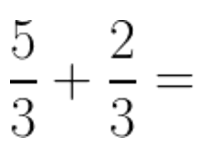The only thing we need to do is add the numerators and leave the denominator alone. The answer is: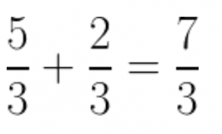### Adding Numbers and a Fraction

For example: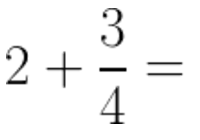The first thing that we need to do in this case is to convert the 2 into a fraction. As you already know, we can simply put a 1 in the denominator of any number without changing its value: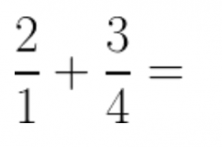When we have two fractions, we can start looking for the common denominator. In this example, it’s quite simple because the least common multiple of 1 and any number is that number. So: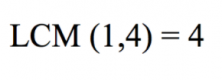Now, we only need to multiply 2 x 4 and we get: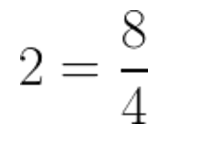…and now we put it into our addition problem: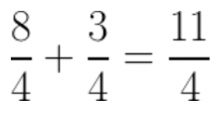### Adding Fractions with Co-Prime Denominators

Remember that two numbers are co-prime if their greatest common divisor is 1. For example, in the problem: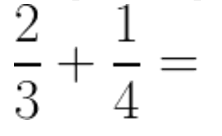The denominators are co-prime because: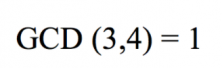These kinds of problems are simple to solve because the only thing that we need to do to find the new numerators is to multiply each numerator by the denominator of the other fraction, as shown below: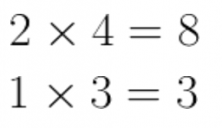And we simply multiply the denominators together. So, we get: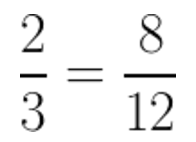and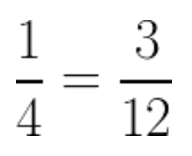And the only thing that’s left to do is to add the two fractions together: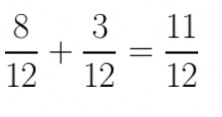For example: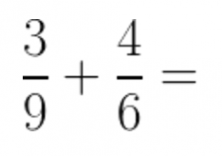We need to calculate the least common multiple of the denominators: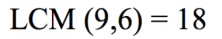What do we have to do next? Let’s break it down. First, let’s look at the fraction: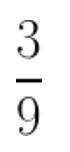To find the numerator, we need to divide the LCM by the fraction’s denominator: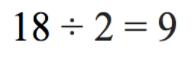We need to multiply the fraction’s numerator by 2. So: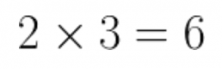And we can see that the new numerator is 6.

For the denominator, we just need to use the GCM (18):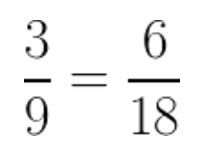Now, we just do the same thing with the other fraction. To solve for the numerator, we need to divide: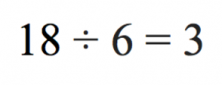And multiply by the numerator: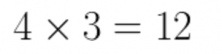Then, we put in the GCM as the denominator, which gives us: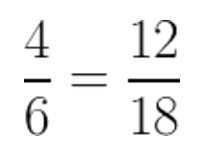Now, all that’s left to do is to add the fractions together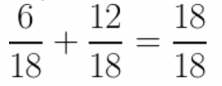And that’s it!

We actually add all fractions this way, the first examples were simpler thanks to the GCM that was easier to work with. The way of solving the problems, however, always stayed the same.

To summarize, the steps for adding fractions are:

• Find the GCM of the two denominators.
• Divide the GCM by the denominator and multiply that by the numerator to convert each fraction into a fraction that has the GCM as the new denominator.
• When we’ve done the two previous steps with all the fractions, put them all in order and add their numerators.

If you want to continue learning math, register today for Smartick!

Good luck adding fractions—with a little practice, you’ll see that they’re a snap and you’ll get it in no time!

Fun is our brain’s favorite way of learning
Diane Ackerman
Smartick is a fun way to learn math
• 15 fun minutes a day
• Millions of students since 2009• man6ySep 11 2021, 2:27 AM

nice

• ValentineApr 25 2021, 4:05 AM

Great

• Amvutia BabelFeb 12 2021, 2:57 PM

• michelleOct 13 2020, 4:51 PM

thank you! it really helps

• Very well ex lainedApr 10 2020, 2:47 PM

Well explained.

• Leonardo messiFeb 27 2019, 5:25 PM

It was helpful and very detailed. My son loves this program and he said it’s very easy to understand. Thank you

• SmartickFeb 28 2019, 5:45 AM

Thank you Leo Messi 😉

Thanks so much for your help

• Emem GabrielFeb 04 2021, 10:50 PM

Well explained, thanks.

• kaylaniSep 20 2018, 8:18 PM

This helped me and my 10 year old daughter in math. THANK YOU SO MUCH!!!!!!